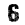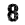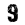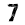# Gain, Loss and Decibels

 Gain x2 +3dB Loss ½ -3dB Gain x 4 +6dB Loss ¼ -6dB Gain x 10 +10dB Loss 1/10 -10dB Gain x 100 +20dB Loss 1/100 -20dB Gain x 1000 +30dB Loss 1/1000 -30dB Gain x 10000 +40dB Loss 1/10000 -40dB

Example 400Watt = 4 x 100 watts   = + 6dB + 20dB = +26dBW

1000watts = +10dB + 100dB = +30dBW

Amplifiers and Antennas produce a positive gain in +dB

Feeders, filters and attenuators produce a negative gain or loss –dB

Antenna gains are quoted in two quantities which need to be understood.

dBi = Gain in reference to a isotropic antenna (A ideal theoretical antenna of no use in practise) .

dBD = Gain in reference to a dipole

dBD= dBi gain – 2.15

The most practical unit is dBD since the effective radiated power erp = power x gain in dBD.

e.g   A Beam antenna of 10dBd gain is fed with 100 watts what is the erp = 10 x 100

= 1000 watts.

If a similar antenna gain was quoted as 10dBi then the erp would be much reduced since dBD = dBi-2.15 = 7.05dBD and erp = 7.05 x 100 = 700.35 watts.

Some manufactures quote dBi instead of dBD because it is a higher numerical value of gain and it looks like you are getting more for your money, but you are not.

Actual useful gain on 2m = (dBi – 2.15) = 7.5-2.15 = 5.35 dBD and on 70cms = 7.15dBD

Simple HF dipole

A simple dipole can be made for any band but it is best to use a half wave of the lowest band possible and the higher bands with are multiples of half wavelength can also be used.Open feeder or 300Ω – Ribbon cable direct to balanced input on ATU